# Extracting Integers and Fractions in Microsoft Excel

Sometimes you need to extract the integer portion of a number. Sometimes the fractional part. Sometimes both. Excel makes it easy to get the integer and somewhat harder to get the fraction. If you just want the answer, skip to the technical details.

### The Integer Part: Excel INT Function

What could be easier than the Excel INT function? I mean INT almost screams INTEGER. So the name is intuitive. You almost “know” what it’s going to do, even if you haven’t used it before.

With only one argument, it’s execution is even simpler. Just feed it a number and … Read the rest# Extract Time with the MOD Function in Excel

I had a reader comment on my last post about how to extract time from a date-time number using the MOD function. Simple really.

The syntax is MOD(number,divisor). The MOD function returns the remainder after number is divided by divisor. A simple example is MOD(5,2), which equals one (1). It works like this: five (5) divided by two (2) equals two (2), with one (1) left over.

All numbers are evenly divisible by one (1) so the MOD function returns any fractional part when the second argument is one (1).

In the screen shot below, cell C2 has … Read the rest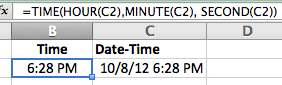# Extract Time from a Date-Time Number in Excel

I have a worksheet that tracks start and stop times for different events throughout the day, all during the week. Sometimes I have to pull out the Time of Day, irrespective of the Date, with the TIME function.

The TIME function has three arguments: Hour, Minute, Second. I could use =TIME(11,30,0) in a cell to get 11:30 AM, but I want to convert a Date-Time number so let’s look at an example.

In cell C2 I have the Date-Time value 10/8/12 6:28:30 PM.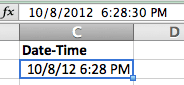In cell B2 I enter the formula =TIME(HOUR(C2),MINUTE(C2), SECOND(C2)) to pull out the time value. … Read the rest

# Extract a Date from a Date-Time Number in Excel

I recently saw a spreadsheet with the following function =LEFT(A2,8) where cell A2 was equal to 08/06/12 12:23 PM. The user was trying to extract the Date from the Date-Time value. The problem for me was that the LEFT function returns a “text” value. Excel is good at recognizing text values that look like dates, as Dates, but why not use a formula that returns a numeric value?

My reflex was to enter the following formula =DATE(YEAR(A2),MONTH(A2),DAY(A2)) and get a proper Date value. You can tell the difference because, with no cell formatting, a Text value is left-justified … Read the rest

# Pay Periods and Funky PivotTable Controls

I like to use a PivotTable to figure out simple problems in Excel. So for this post I’m going to use Excel 2011 (Mac), where PivotTable controls look funky when compared to their Windows counterpart.

Since I get paid every two weeks, certain months in a year will contain three pay periods. Planning future vacations during these months isn’t a bad idea, so I’m going to look at pay periods for the next three years.

### Add a Column of Dates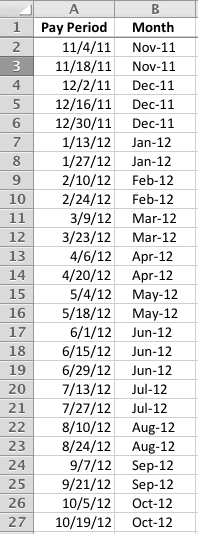I’ll enter the first pay period, then create a formula that adds 14 days and copy it down to get … Read the rest

# International Short Date Formatting for the TEXT Function

I have an Excel workbook that is used in two different regions where the date format is entirely different, the US and the UK. At the top of a report worksheet I use a TEXT function to inform the user of the date range.

Here’s what a US user sees:

From: 6/6/2011 to 6/10/2011

Here is the formula:

=”From: ” & TEXT(MIN(ExtractData!A:A),”m/d/yyyy”) & ” to ” & TEXT(MAX(ExtractData!A:A),”m/d/yyyy”)

The Min and Max dates are in column A on a worksheet named ExtractData.

The problem is trying to automatically change the date format in the second argument of the TEXT Function … Read the rest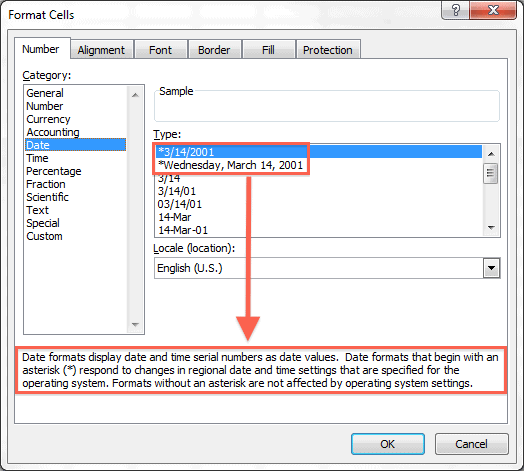# Regional Date Formats in Excel

I recently returned from two weeks in Ireland where I installed a couple of Excel reporting systems and came across the dreaded regional date settings. My computer is set for the US region, whereas the client’s computers were set for the UK region. When I mention regional settings on my computer, I’m talking about Windows and not Excel.

Trying to get Excel to automatically switch date formats between computers using different region settings was something I experienced a few years ago while working in England. Back then I made the mistake of ustng Excel to change the formatting for … Read the rest

# Quickly Adjust 1904 Dates for the 1900 Date System in Excel

An associate of mine “helped” me out once by putting a worksheet together for data entry. After the worksheet had several days of user input, I found out the workbook was created on a Mac with an older version of Excel for Mac (2004) that defaulted to the 1904 date system.

Since the worksheet in question would be used entirely by Windows users, I decided to change to the 1900 date system to avoid any problems. Little did I know that by changing that simple date system setting would change the dates by 1462 days.

That’s because first day of … Read the rest

# A Woopra Chat About ExcelI use the Woopra desktop app to view live visitors to this WordPress blog. Visitors will see a Woopra window lurking about when I’m logged into my (free) Woopra account. One option is to initiate a chat session with me.

My first chat happened the other day. The chat notice startled me, but I quickly recovered and was able to interact with a visitor.

(Bold and color are my additions for clarity)

Visitor #13357:
08:53:19. HI
You:
08:53:28. Howdy

Visitor #13357:
08:54:22. I WANT TO CONVERT 01:05 = 65 MIN WHAT I DO
08:55:42. IN EXCEL
You:
08:56:58. In cell Read the rest

# Let Excel Convert Hours Between Two Dates and Times

In a previous post I went on and on about how you can calculate hours between two different dates and times, but Excel does this for you automatically. I’ll use reader comment as my example.

A-02.02.2011 10:00
B-05.02.2011 17:00

For clarity I will assume that the date format is m/d/yyyy, which is what I use here in the US.

For the A value type in 2/2/2011 10:00 and for the B value type in 5/2/2011 17:00 then in another cell subtract A from B. In the (B-A) cell enter the custom time … Read the rest

# What Day of the Year is It?

Given the Date in this spreadsheet, a simple formula using the DATE Function will return the Day of Year. Go ahead and type a Date value in cell A2 to see how the formula works, the spreadsheet is embedded from my SkyDrive. (Date format is US, “m/d/yyyy”)

## The Day of Year Formula

The formula in cell B2 is:

= A2-DATE(YEAR(A2),1,0)

The DATE Function takes the Year from the Date you type in cell A2, and uses the zero (0) day of month 1 (January), which is the last day … Read the rest

# How Many Years, Months and Days Has it Been?

The DATEDIF Function comes in handy when you want to know how many years, months, and days there are between two dates. And even though it may be slightly flawed, it still beats the alternatives.

The following spreadsheet works well for birthdays or anniversaries. The DATEDIF Function is being used to generate the Years, Months, and Days.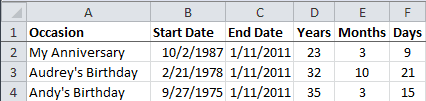The End Date column has the formula =TODAY().

The Years heading in cell D2 has the custom format ;;;”Years” which shows the text “Years” in the spreadsheet cell while hiding the underlying value “y” that you can see in the formula bar.… Read the rest

# Calculate Hours Between Two Dates and Times in Excel

Recently I was asked how to subtract time in Excel (time difference) or how to calculate the number of hours between two points in time on different days. Since this was in a reader comment, I gave a brief answer that requires a fuller account here.

Dates and Times are all part of the master plan in Excel. Once you “get” the fundamentals, the rest is just icing on the cake.

A Date value in Excel looks like this: 40519
A Time value in Excel looks like this: 0.58333

Cell formatting changes how you see these numbers.

The Date: 7 … Read the rest

# Calculate the Xth Weekday of Any Month in Excel

I’ve a simple formula for calculating the Xth Weekday of ANY month. It takes four inputs: Year, Month, Week, and Day. And requires a couple of lookup tables for data validation, one with special formatting.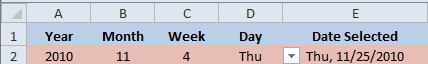### The History

It all started when my wife mentioned there were a couple of meetings she had to schedule at work, one on the second Tuesday of every month and the other on the third Tuesday of every month. That got me to thinking about how to create a formula in Excel to calculate those dates.

After getting a partial solution, other things … Read the rest

# Convert Pace to MPH and Back Again in Excel

Excel is a great tool for figuring stuff out, like for conversions that aren’t easy to do in your head. Here I’m converting Pace to MPH and then reversing the process, converting MPH to Pace, to create a conversion chart.

## My Conversion Problem for Minutes per Mile to MPH

I track my Average Pace when out walking for exercise by using the iPhone App Walkmeter, then log that information into the Lose It App. The problem I have is converting my Average Pace to Miles per Hour (MPH).

Below you can see my average pace is 13:22 per mile, … Read the rest